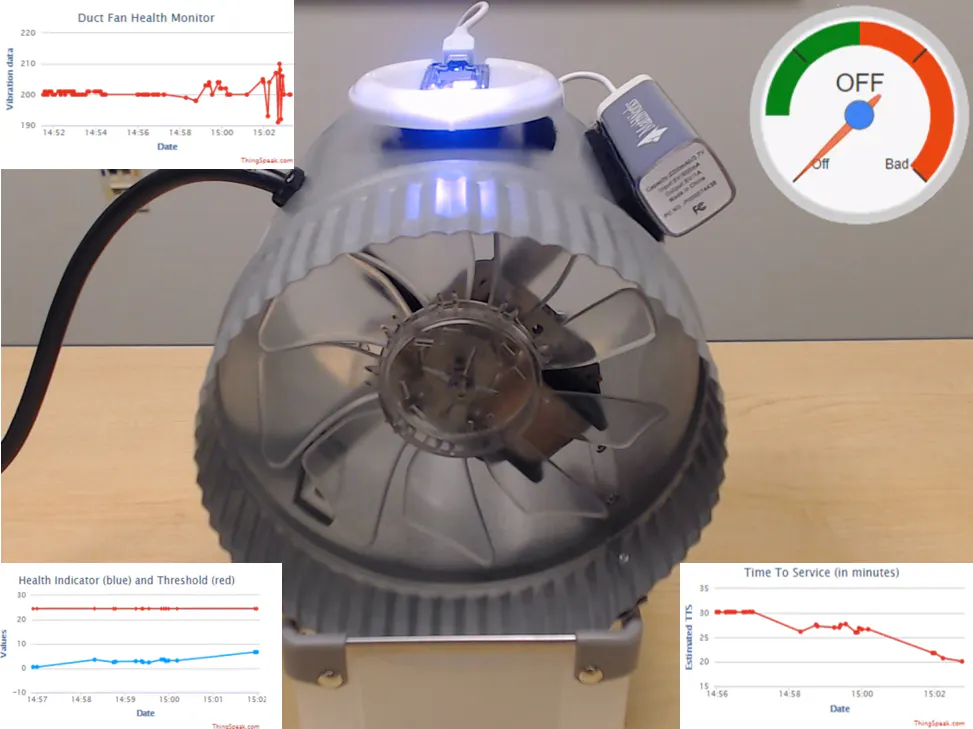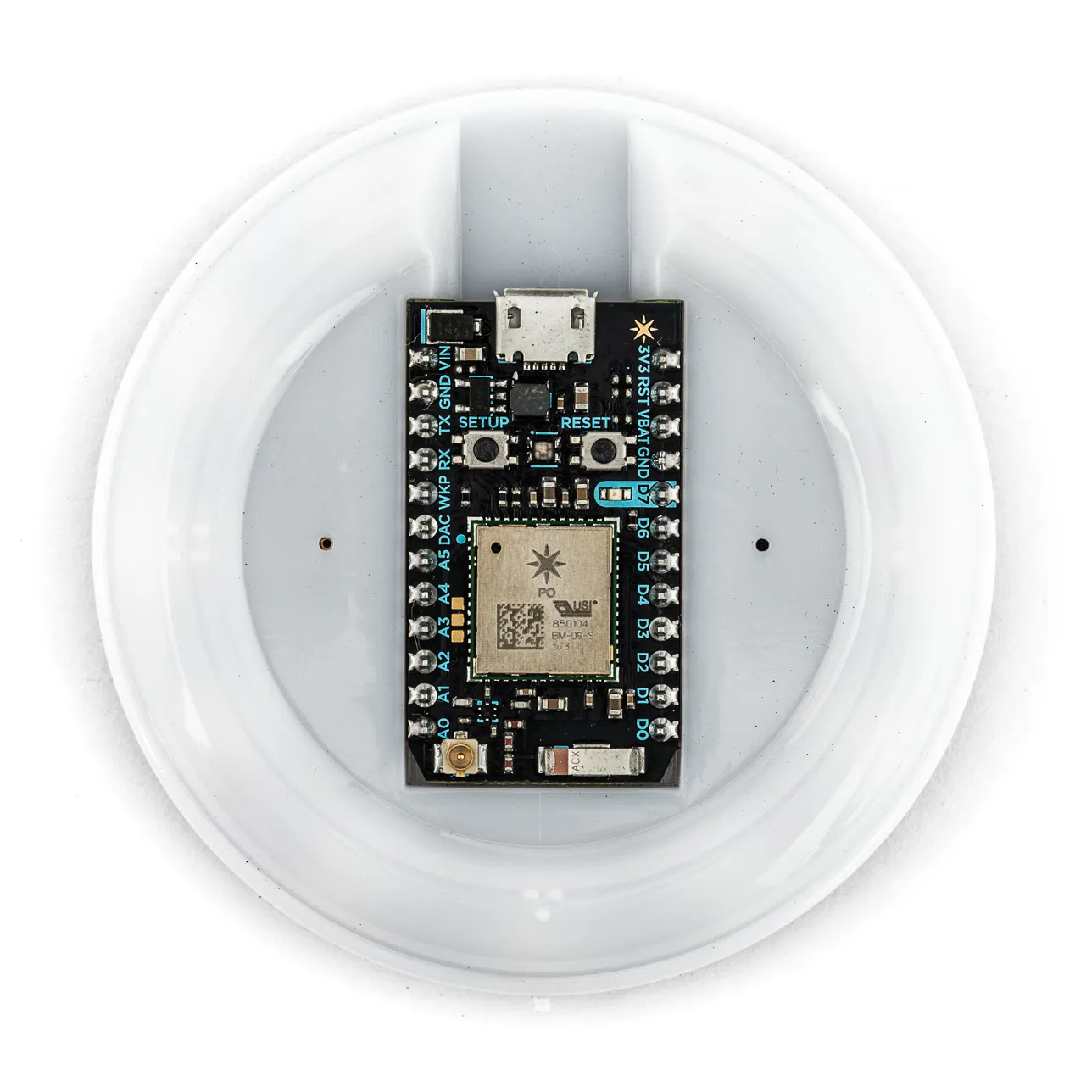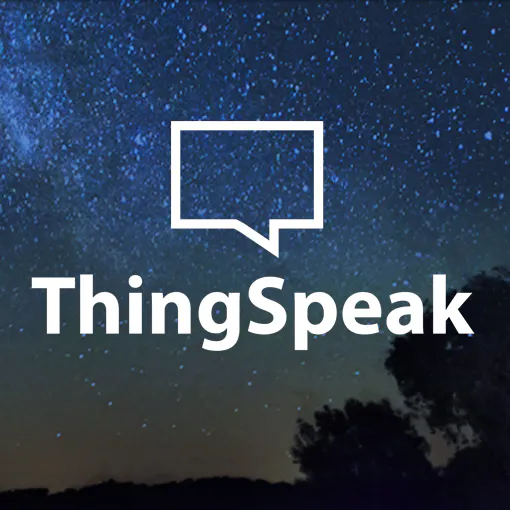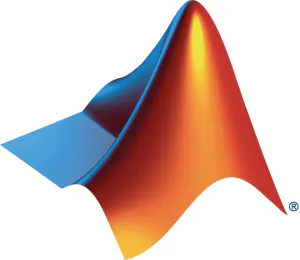# Condition-based Maintenance of a Duct Fan using ThingSpeak

An example of a condition-based maintenance system using algorithms in MATLAB to identify faults & estimate time to service.

IntermediateFull instructions provided5 hours5,479## Things used in this project

### Hardware componentsParticle Internet Button
×1
 Duct Fan Any similar equipment can work with appropriate training of the algorithm.
×1

### Software apps and online servicesThingSpeak APIMATLABIFTTT Maker service

### Hand tools and fabrication machines

 Velcro
 Vise
 Power source
 USB Power Bank
 Cardboard
 Duct Tape

## Code

### Training.m

MATLAB
```%%
%Data Collection and Labeling
clc;
clear all;
labelPoints = {[252,500],[251,500]}; %FILL IN THE LABEL POINTS eg. {[252,500],[251,500]}
NoOfFailureModes = 2;
estimateRULFlags = [false, true]; %change the corresponding flags to enable/disable RUL estimation for corresponding failure modes
path = 'Datasets/'; %Verify if this is the correct path for your dataset files
labels = {[categorical("OFF"),categorical("ON"),categorical("DUCT BLOCKAGE")], ...
[categorical("OFF"),categorical("ON"),categorical("ROTOR IMBALANCE")]};

rawdata = cell(NoOfFailureModes);
data = cell(NoOfFailureModes);

for i = 1:NoOfFailureModes
data{i} = dataParsing(rawdata{i},labelPoints{i},labels{i},i); %Parse the rawdata
end

%%
%Data Exploration
fs = 100; %Sampling Frequency
L = 100; %Length of each data sample is 100 sensor readings
f = fs*(0:(L/2))/L; % Frequency bins until Nyquist Frequency at which FFT is computed
convertToMinutes = (1/fs)/60; %Convert sample number to minutes 0.01/60
votype = 'VideoWriter'; %Record the Exploration to view it again
vo = VideoWriter('Data Exploration Video', 'MPEG-4');
set(vo, 'FrameRate', 5);
open(vo);
for i = 1:NoOfFailureModes
NoOfDataPoints = height(data{i});
x = zeros(L*NoOfDataPoints,0);
y = zeros(L*NoOfDataPoints,0);
z = zeros(L*NoOfDataPoints,0);
figure;
set(gcf,'Visible','on')
for j = 1:NoOfDataPoints
data{i}.X_FFT(j,:) = computeFFT(data{i}.X(j,:)-mean(data{i}.X(j,:)),L);
data{i}.Y_FFT(j,:) = computeFFT(data{i}.Y(j,:)-mean(data{i}.Y(j,:)),L);
data{i}.Z_FFT(j,:) = computeFFT(data{i}.Z(j,:)-mean(data{i}.Z(j,:)),L);
x((j-1)*L+1 : j*L) = data{i}.X(j,:);
y((j-1)*L+1 : j*L) = data{i}.Y(j,:);
z((j-1)*L+1 : j*L) = data{i}.Z(j,:);
t = (1:j*L)*convertToMinutes;

subplot(3,2,1);
plot(t,x);
title({"Experiment: " + num2str(i) , "Time Domain"});
xlabel("time (min.)",'FontWeight','bold');
ylabel("X",'rotation',0,'FontWeight','bold');

subplot(3,2,2);
bar(f,data{i}.X_FFT(j,:));
title({"Sample: " + num2str(j) + "; Label: " + char(data{i}.Label(j)), "Frequency Domain"});
xlabel("frequency (Hz)",'FontWeight','bold');
ylabel("X",'rotation',0,'FontWeight','bold');

subplot(3,2,3);
plot(t,y);
xlabel("time (min.)",'FontWeight','bold');
ylabel("Y",'rotation',0,'FontWeight','bold');

subplot(3,2,4);
bar(f,data{i}.Y_FFT(j,:));
xlabel("frequency (Hz)",'FontWeight','bold');
ylabel("Y",'rotation',0,'FontWeight','bold');

subplot(3,2,5);
plot(t,z);
xlabel("time (min.)",'FontWeight','bold');
ylabel("Z",'rotation',0,'FontWeight','bold');

subplot(3,2,6);
bar(f,data{i}.Z_FFT(j,:));
xlabel("frequency (Hz)",'FontWeight','bold');
ylabel("Z",'rotation',0,'FontWeight','bold');

drawnow;
writeVideo(vo, getframe(gcf));
end
end
close(vo);
%%
%Feature Extraction
combinedFeatures = cell2table(cell(0,0));
features = cell(NoOfFailureModes);
for i = 1:NoOfFailureModes %Combine the experiments data in one variable
features{i} = extractFeatures(data{i});
combinedFeatures = [combinedFeatures;features{i}];
end

combinedFeatures = sortrows(combinedFeatures); %Sort the combined data based on Label
combinedFeatures.sno = []; %Delete sno, it is no longer needed after sorting.
NoOfDataPoints = width(combinedFeatures);
for i = 1:NoOfDataPoints %Plot the features in different figures
if(mod(i-1,4)==0)
figure;
end
subplot(4,1,mod(i-1,4)+1);
plot(combinedFeatures.(i));
xlabel('Sample No.');
ylabel(combinedFeatures.Properties.VariableNames(i));
end

%%
%Build Machine Learning Model
save('Fan Diagonizer Model.mat','trainedModel','labels');
%%
%Synthesize Health Indicator
selectedFeatures = cell(NoOfFailureModes,1);
selectedFeatures{1,:} = {'FreqX','FreqY'}; %FILL IN the selected features for Experiment 1 if you estimate RUL for failure mode 1 eg. {'FreqX','FreqY'}
selectedFeatures{2,:} = {'StdZ'}; %FILL IN the selected features for Experiment 2 if you estimate RUL for failure mode 2 eg. {'StdZ'}
newFeatures = features;
meanTrain = cell(NoOfFailureModes,1);
sdTrain = cell(NoOfFailureModes,1);
pcaCoeff = cell(NoOfFailureModes,1);
healthIndicator = cell(NoOfFailureModes,1);
conversion = cell(NoOfFailureModes,1);
time = cell(NoOfFailureModes,1);
for i = 1:NoOfFailureModes
if(estimateRULFlags(i))
newFeatures{i}(newFeatures{i}.Label == 'OFF',:) = []; % Delete OFF data as it does not depend on Fan's health
conversion{i} = getAvgSampleTime(rawdata{i}.created_at)/60; %in get average sample time in minutes from ThingSpeak time stamp
newFeatures{i} = newFeatures{i}(:,selectedFeatures{i,:}); %Choose the selected features
if(length(selectedFeatures{i,:}) ~= 1) %If there are more than 1 feature, fuse them using Principle Component Analysis
meanTrain{i} = mean(newFeatures{i}{:,:});
sdTrain{i} = std(newFeatures{i}{:,:});
DataNormalized = (newFeatures{i}{:,:} - meanTrain{i})./sdTrain{i};
pcaCoeff{i} = pca(DataNormalized);
healthIndicator{i} = (newFeatures{i}{:,:} - meanTrain{i}) ./ sdTrain{i} * pcaCoeff{i}(:, 1);
healthIndicator{i} = healthIndicator{i}(126:end); %Collect same number of data points from On state as in each failure level (125 points)
else
meanTrain{i} = NaN;
sdTrain{i} = NaN;
pcaCoeff{i} = NaN;
healthIndicator{i} = newFeatures{i}{:,:};
healthIndicator{i} = healthIndicator{i}(126:end);
end
figure;
time{i} = (1:length(healthIndicator{i}));
plot(time{i},healthIndicator{i},'-o');
title(['Experiment ' num2str(i)]);
xlabel('time (in min.)');
ylabel('Health Indicator');
end
end
%%
%Build and Fit RUL model
mdl = cell(NoOfFailureModes,1);
for i = 1:NoOfFailureModes
if(estimateRULFlags(i))
if(healthIndicator{i}(1) > healthIndicator{i}(end)) % if health indicator is showing decreasing trend
threshold{1} = min(healthIndicator{1}); % take the least value in the experiment as threshold
yIntercept{1} = 1e7;  %An arbitrarily large value to initialize the model
else
threshold{2} = max(healthIndicator{2}); % take the highest value in the experiment as threshold
yIntercept{2} = -1e7; %An arbitrarily large value to initialize the model
end
'Theta', 0, ...
'ThetaVariance', 1e-5, ...
'Phi',  yIntercept{i}, ...
'NoiseVariance', (0.1*threshold{i}/(threshold{i} + 1))^2, ... Assume 10% noise at the threshold
'SlopeDetectionLevel', []);

mdl{i}.fit({[(1:length(healthIndicator{i}))' healthIndicator{i}]}); %Fit the model

totalLength = length(healthIndicator{i}) - 1;
estTTSs = zeros(totalLength, 1);
trueTTSs = zeros(totalLength, 1);
CITTSs = zeros(totalLength, 2);
pdfTTSs = cell(totalLength, 1);
timeUnit = 'minutes';

% Create figures and axes for plot updating
figure
ax1 = subplot(2, 1, 1);
ax2 = subplot(2, 1, 2);
for currentLength = 1:totalLength
% Predict Remaining Useful Life
[estTTS, CITTS, pdfTTS] = predictRUL(mdl{i},[currentLength healthIndicator{i}(currentLength)],threshold{i});
trueTTS = (totalLength - currentLength + 1);
% Keep prediction results
estTTSs(currentLength) = estTTS;
trueTTSs(currentLength) = trueTTS;
CITTSs(currentLength, :) = CITTS;
pdfTTSs{currentLength} = pdfTTS;
end
helperPlotTrend(ax1, currentLength, healthIndicator{i}, mdl{i}, threshold{i}, timeUnit,conversion{i});
plot(ax2,(1:totalLength)*conversion{i},estTTSs*conversion{i});
xlabel('Time (minutes)');
ylabel('Time to Service (minutes)');
end
end
save('Fan TTS Model.mat','mdl','conversion','threshold', 'meanTrain', 'sdTrain','pcaCoeff','selectedFeatures');
%%
%Helper Functions
function P1 = computeFFT(X,L)
Y = fft(X);
P2 = abs(Y/L);
P1 = P2(1:L/2+1);
P1(2:end-1) = 2*P1(2:end-1);
end

function avgTime = getAvgSampleTime(data)
x = cellfun(@decode,data);
for i = 1:length(x)
y(i,:) = datevec(x(i));
end
for i = 2:length(y)
diff(i,:) = etime(y(i,:),y(i-1,:));
end
avgTime = mean(diff); %in seconds
end

function y = decode(data)
y = (datetime(data,'TimeZone','local','Format','yyyy-MM-dd HH:mm:ss Z'));
end

function helperPlotTrend(ax, currentLength, healthIndicator, mdl, threshold, timeUnit, conversion)
t = (1:size(healthIndicator, 1))*conversion;
HIpred = mdl.Phi + mdl.Theta*t/conversion;
title(ax, sprintf('Sample %d', currentLength));
cla(ax)
hold(ax,'on');
plot(ax, t, HIpred);
plot(ax, t(1:currentLength), healthIndicator(1:currentLength, :))
plot(ax, t, threshold*ones(1, length(t)), 'r')
ylabel(ax, 'Health Indicator')
xlabel(ax, ['Time (' timeUnit ')'])
legend(ax, 'Model','Health Indicator', 'Threshold', 'Location', 'Northwest')
end

function data = dataParsing(rawdata,labelPoints,labels,dataset)
bias = 128;
noOfDataPoints = length(rawdata.entry_id);
labelPoints = [0 labelPoints noOfDataPoints];

for i = 1:length(labelPoints)-1
Label(labelPoints(i)+1:labelPoints(i+1),:) = labels(i); %Create Labels for the data points
end
j = 1;

if(dataset == 2) %offset for sno for the second experiment, to avoid same sno for the data points between experiments
offset = 0;
else
offset = 2000;
end

for i = 1:noOfDataPoints
text1 = strsplit(rawdata.field1{i},',');
text2 = strsplit(rawdata.field2{i},',');
text3 = strsplit(rawdata.field3{i},',');
text4 = strsplit(rawdata.field4{i},',');
text5 = strsplit(rawdata.field5{i},',');
text6 = strsplit(rawdata.field6{i},',');
var = zeros(length(text1)-1,6);
flag = true;
for k = 1:length(text1)-1 %to neglect the last comma, use -1
var(k,1) = str2num(text1{k})-bias;
var(k,2) = str2num(text2{k})-bias;
var(k,3) = str2num(text3{k})-bias;
if(var(k,1) ~= -bias || var(k,2) ~= -bias || var(k,3) ~= -bias ) %Turning the device off and on will reset the first data set to all zeroes
var(k,4) = str2num(text4{k})-bias;
var(k,5) = str2num(text5{k})-bias;
var(k,6) = str2num(text6{k})-bias;
else
flag = false;
break;
end
end
if(flag) %accept a valid datapoint
data.Label(j,:) = Label(i);
data.sno(j,:) = rawdata.entry_id(i) + offset;
data.X(j,:) = [var(:,1);var(:,4)];
data.Y(j,:) = [var(:,2);var(:,5)];
data.Z(j,:) = [var(:,3);var(:,6)];
j = j+1;
end
end

data = struct2table(data);

end

function features = extractFeatures (data)
features.Label = data.Label;
features.sno = data.sno;
freqN = 0; %No. of top frequency bins to compute cumulative energy

fs = 100; %Sampling Frequency in Hz
L = 100; %No. of sensor readings in each data point
f = fs*(0:(L/2))/L; %Frequencies (till Nyquist frequency) at which FFT is computed
for i = 1:height(data)
signalX = data.X(i,:);
features.StdX(i,:) = std(signalX);
signalX = (signalX - mean(data.X(i,:)))/features.StdX(i,:);
signalX_FFT = sortrows([f;computeFFT(signalX,L)]',2,'descend');
features.FreqX(i,:) = signalX_FFT(1,1);
features.EnergyAtFreqX(i,:) = sum(signalX_FFT(1:1+freqN,2));

signalY = data.Y(i,:);
features.StdY(i,:) = std(signalY);
signalY = (signalY - mean(data.Y(i,:)))/features.StdY(i,:);
signalY_FFT = sortrows([f;computeFFT(signalY,L)]',2,'descend');
features.FreqY(i,:) = signalY_FFT(1,1);
features.EnergyAtFreqY(i,:) = sum(signalY_FFT(1:1+freqN,2));

signalZ = data.Z(i,:);
features.StdZ(i,:) = std(signalZ);
signalZ = (signalZ - mean(data.Z(i,:)))/features.StdZ(i,:);
signalZ_FFT = sortrows([f;computeFFT(signalZ,L)]',2,'descend');
features.FreqZ(i,:) = signalZ_FFT(1,1);
features.EnergyAtFreqZ(i,:) = sum(signalZ_FFT(1:1+freqN,2));

end
features = struct2table(features);
end
```

### Analysis.m

MATLAB
```clc;
clear all;
%Fill in the following values
firstChID = 111111; %FILL IN first channel's ID
secondChID = 123456;  %FILL IN second channel's ID
secondWriteAPIKey = 'YYYYYYYYYYYYYYYY'; %FILL IN second channel's Write API key
IFTTTURL = 'https://maker.ifttt.com/trigger/Alert/with/key/1a1a1a1a1a1a1a1a1a1a1'; %FILL IN IFTTT URL
deviceID = '123456789012345678901234567890'; %FILL IN internet button's device ID
thresholdTTS = 10; %email will be sent if the fan's TTS is less than this value (in minutes)
%%%%%%%%%%%%%%%%%%%%%%%%%%%%%%%%%%%%%%%%%%%%%%%%%%%%%%%%%%%%%%%%%%
bias = 128;
data = dataParsing(rawdata,bias); %Parse the six string fields
features = extractFeatures(data); %Extract features from this data
[mdl, labels] = getmodel(dropBoxAccessToken); %Get the ML Classifier model from DropBox
fanState = (mdl.predictFcn(features)); %Predict the state of the Fan
display(fanState,'Output');
switch(fanState)
case labels{1}(1)
out = 0;
case labels{1}(2)
out = 1;
case labels{1}(3)
out = 2;
case labels{2}(3)
out = 3;
end
getValues(features, out,dropBoxAccessToken, IFTTTURL, secondChID,thresholdTTS,labels,secondReadAPIKey) %Get the others values to be written on second channel

'Fields',[1,2,3,4,5,6],'WriteKey',secondWriteAPIKey); %Write the results in second channel
try
catch someException
display(someException.message);
end

if(out == 0 || out == 1) %Don't need Predictive Maintenance during normal operation
estTTS = NaN;
healthIndicator = NaN;
threshold = NaN;
else
f = fopen('Fan TTS Model.mat','w');
fwrite(f,rawdata);
fclose(f);
failureMode = out - 1;
if(length(T.selectedFeatures{failureMode})==1)
healthIndicator =  table2array(features(:,T.selectedFeatures{failureMode}));
threshold = T.threshold{failureMode};
[estTTS, CITTS, pdfTTS] = predictRUL(T.mdl{failureMode},[-NaN healthIndicator],threshold);
estTTS = estTTS*T.conversion{failureMode};
CITTS = CITTS*T.conversion{failureMode};
pdfTTS.TTS = pdfTTS.RUL*T.conversion{failureMode};
else
selectedFeatures = features(:,T.selectedFeatures{failureMode});
healthIndicator = (selectedFeatures{:,:} - T.meanTrain{failureMode}) ./ T.sdTrain{failureMode} * T.pcaCoeff{failureMode}(:, 1);
threshold = T.threshold{failureMode};
[estTTS, CITTS, pdfTTS] = predictRUL(T.mdl{failureMode},[-NaN healthIndicator],threshold);
estTTS = estTTS*T.conversion{failureMode};
CITTS = CITTS*T.conversion{failureMode};
pdfTTS.TTS = pdfTTS.RUL*T.conversion{failureMode};
end

end

if(~isstruct(Flag))
else
if(out == 3 && estTTS < thresholdTTS && Flag.field6 == '0') %if the email hasn't been sent and if the Fan needs a maintanenance
response = webwrite(IFTTTURL,'value1', string(estTTS)); %Request IFTTT service to send the email notification
EmailAlertFlag = 1; %set the flag to avoid multiple emails.
else
if(Flag.field6 == '1' && out == 1) %if there was an email sent before and fan returns to normal operation indicating the performance of maintanenance
EmailAlertFlag = 0; %Reset the flag
else
end
end
end
end

function data = dataParsing(rawdata,bias)
noOfDataPoints = length(rawdata.Timestamps);
for i = 1:noOfDataPoints
text1 = strsplit(rawdata.X1{i},',');
text2 = strsplit(rawdata.Y1{i},',');
text3 = strsplit(rawdata.Z1{i},',');
text4 = strsplit(rawdata.X2{i},',');
text5 = strsplit(rawdata.Y2{i},',');
text6 = strsplit(rawdata.Z2{i},',');
var = zeros(length(text1)-1,6);
for k = 1:length(text1)-1 %to neglect the last comma, use -1
var(k,1) = str2num(text1{k})-bias;
var(k,2) = str2num(text2{k})-bias;
var(k,3) = str2num(text3{k})-bias;
var(k,4) = str2num(text4{k})-bias;
var(k,5) = str2num(text5{k})-bias;
var(k,6) = str2num(text6{k})-bias;
end
data.X(i,:) = [var(:,1);var(:,4)];
data.Y(i,:) = [var(:,2);var(:,5)];
data.Z(i,:) = [var(:,3);var(:,6)];
end
data = struct2table(data);
end

function features = extractFeatures(data)
freqN = 0; %No. of top frequency bins to compute cumulative energy

fs = 100; %Sampling Frequency in Hz
L = 100; %No. of sensor readings in each data point
f = fs*(0:(L/2))/L; %Frequencies (till Nyquist frequency) at which FFT is computed
for i = 1:height(data)
signalX = data.X(i,:);
features.StdX(i,:) = std(signalX);
signalX = (signalX - mean(data.X(i,:)))/features.StdX(i,:);
signalX_FFT = sortrows([f;computeFFT(signalX,L)]',2,'descend');
features.FreqX(i,:) = signalX_FFT(1,1);
features.EnergyAtFreqX(i,:) = sum(signalX_FFT(1:1+freqN,2));

signalY = data.Y(i,:);
features.StdY(i,:) = std(signalY);
signalY = (signalY - mean(data.Y(i,:)))/features.StdY(i,:);
signalY_FFT = sortrows([f;computeFFT(signalY,L)]',2,'descend');
features.FreqY(i,:) = signalY_FFT(1,1);
features.EnergyAtFreqY(i,:) = sum(signalY_FFT(1:1+freqN,2));

signalZ = data.Z(i,:);
features.StdZ(i,:) = std(signalZ);
signalZ = (signalZ - mean(data.Z(i,:)))/features.StdZ(i,:);
signalZ_FFT = sortrows([f;computeFFT(signalZ,L)]',2,'descend');
features.FreqZ(i,:) = signalZ_FFT(1,1);
features.EnergyAtFreqZ(i,:) = sum(signalZ_FFT(1:1+freqN,2));

end
features = struct2table(features);
end

function P1 = computeFFT(X,L)
Y = fft(X);
P2 = abs(Y/L);
P1 = P2(1:L/2+1);
P1(2:end-1) = 2*P1(2:end-1);
end

function [mdl,labels] = getmodel(dropBoxAccessToken)
f = fopen('Fan Diagonizer Model.mat','w');
fwrite(f,rawdata);
fclose(f);
thefile = matfile('Fan Diagonizer Model.mat');
mdl = thefile.trainedModel;
labels = thefile.labels;
end

narginchk(1,2);

FName = varargin{1};

headerFields = {'Authorization', ['Bearer ', dropboxAccessToken]};

% Set the options for WEBREAD
opt = weboptions;
opt.MediaType = 'application/octet-stream';
opt.CharacterEncoding = 'ISO-8859-1';
opt.RequestMethod = 'post';

try
catch someException
end

% If user requested output, pass along WEBWRITE output
if isequal(nargout,1)
output = tempOutput;
end
end

% Check if input file exists
if ~exist(dataFile,'file')
end

try
fid = fopen(dataFile, 'r');
fclose(fid);
catch someException
end

[~,remoteFName, remoteExt] = fileparts(dataFile);
headerFields = {'Authorization', ['Bearer ', dropboxAccessToken]};
headerFields{2,2} = sprintf('{"path": "/%s%s", "mode": "overwrite", "autorename": false, "mute": false}',remoteFName, remoteExt);

% Set the options for WEBWRITE
opt = weboptions;
opt.MediaType = 'application/octet-stream';
opt.CharacterEncoding = 'ISO-8859-1';
opt.RequestMethod = 'post';

try
catch someException
end

% If user requested output, pass along WEBWRITE output
if isequal(nargout,1)
output = tempOutput;
end
end
```

### Gauge.js

JavaScript
```<script type='text/javascript' src='https://ajax.googleapis.com/ajax/libs/jquery/1.9.1/jquery.min.js'></script>
<script type='text/javascript'>

// set your channel id here
var channel_id = 123456;
var api_key = 'ZZZZZZZZZZZZZZZZ';
// list of name off the gauge and value
var value_names = ['','ON','BLOCK','TAPE'];

// global variables
var chart, charts, data;

// display the data
function displayData(point) {
data.setValue(0, 0, gauge_names[point]);
data.setValue(0, 1, point);
data.setFormattedValue(0, 1, value_names[point]);    //Formatting font and font size is not possible in gauge chart
chart.draw(data, options);
}

// variable for the data point
var p;

// get the data from thingspeak
\$.getJSON('https://api.thingspeak.com/channels/' + channel_id + '/feed/last.json?api_key=' + api_key, function(data) {

// get the data point
p = data.field3;

// if there is a data point display it
if (p) {
displayData(p);
}

});
}

// initialize the chart
function initChart() {

options = {width: 240, height: 240, max: 3, redFrom: 1.5, redTo: 3, greenFrom:0.5, greenTo: 1.5, minorTicks: 0, majorTicks: ["Off","","","Bad"]};

// load new data every 1 second
}

</script>
```

### HealthMonitor.ino

Arduino
```#include <ThingSpeak.h> //You may have to Include these libraries manually in the Build Web IDE
#include <InternetButton.h>

/*Fill in the following values*/
unsigned long myChannelID =  111111;
const char * myWriteAPIKey = "WWWWWWWWWWWWWWWW";

TCPClient client;
const int noOfSamples = 101, bias = 128; //The first reading of the accelerometer in my InternetButton was always corrupted, so I always read 101 readings and drop the first reading
InternetButton button = InternetButton();

Timer myclock(10, do_every_ms); // Timer to sample accelerometer at 100 Hz

int x[noOfSamples], y[noOfSamples], z[noOfSamples], count = -1;
bool ready = true; //Flag that will be set once a set of 100 readings are read from the accelerometer

void setup()
{
button.begin(); //Initialize Internet Button
Particle.function("Alert", setColor); //Setup to call setColor() from the cloud. Particle Device Cloud API calls this function whenever it receives the keyword "Alert" through HTTP POST
Serial.begin(9600); //Initialize Serial port for debugging purposes
ThingSpeak.begin(client); //Initialize ThingSpeak Client
myclock.start(); //Start the timer to read accelerometer data
}

void loop()
{

{
setData(); //Compose CSV texts from the integer readings and set them to their respective fields
sendData(); //Publish the set fields to ThingSpeak Channel
count = -1; //Reset the counter
ready = false; //Reset the flag
}
}

void do_every_ms()
{
if(count == noOfSamples)
{
}
else
{
count++;
x[count] = button.readX() + bias; // Raw sensor readings range from -128 to 128 which can have maximum length
y[count] = button.readY() + bias; // of 4 characters when converted into a String. To have Strings of length
z[count] = button.readZ() + bias; // maximum 3 characters, add a bias term to remove the necessity of a '-' sign.
}
}

void setData()
{

for(int j = 0; j< 2; j++)
{
char text1 = "",text2 = "",text3 = ""; //Each ThingSpeak Field can hold maximum of 255 characters
for(int i = 1 + j*floor(noOfSamples/2); i< (floor(noOfSamples/2)+1)*(1-j) + j*count; i++) //Compose CSV texts from readings
{
strcat(text1, String(x[i])+",");
strcat(text2, String(y[i])+",");
strcat(text3, String(z[i])+",");
}
ThingSpeak.setField(3*j + 1,text1);
ThingSpeak.setField(3*j + 2,text2);
ThingSpeak.setField(3*j + 3,text3);
Serial.printlnf(String(3*j + 1) + ": " + text1);
Serial.printlnf(String(3*j + 2) + ": " + text2);
Serial.printlnf(String(3*j + 3) + ": "  + text3 );
}

}

int setColor(String condition) //Change the color of the LED according to the condition received
{

button.allLedsOff();

if(!strcmp(condition,"0"))
{
button.allLedsOn(0, 0, 0);
}

else if(!strcmp(condition,"1"))
{
button.allLedsOn(0,255, 0); //Green Color
}
else if(!strcmp(condition,"2"))
{
button.allLedsOn(255, 0, 0); //Red Color
}
else if(!strcmp(condition,"3"))
{

button.allLedsOn(255, 0, 0); //Red Color
button.playNote("G6",8); //Alarm
}
else
{
button.allLedsOn(255, 128, 192); //Pink Color
return -1;
}
return 1;
}

void sendData()
{
button.ledOn(6,255, 128, 255); //Turn on the led
Particle.publish(String(ThingSpeak.writeFields(myChannelID, myWriteAPIKey))); //Write the fields on ThingSpeak channel and Publish its response to Particle Console
button.ledOn(6,0,0,0); //Turn off the led
}
```

## Credits

### Giridharan Kumaravelu

1 project • 9 followers

### Hans Scharler

15 projects • 84 followers
IoT Engineer, Maker - I have a toaster that has been tweeting since 2008.